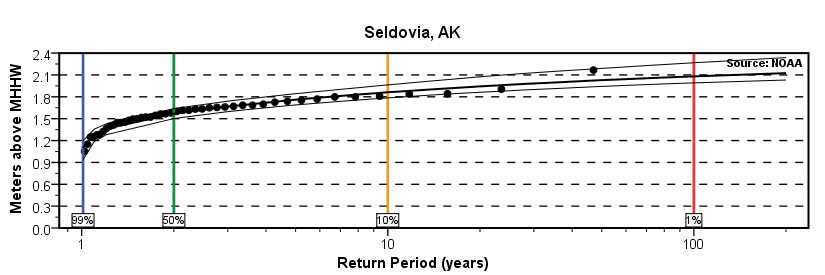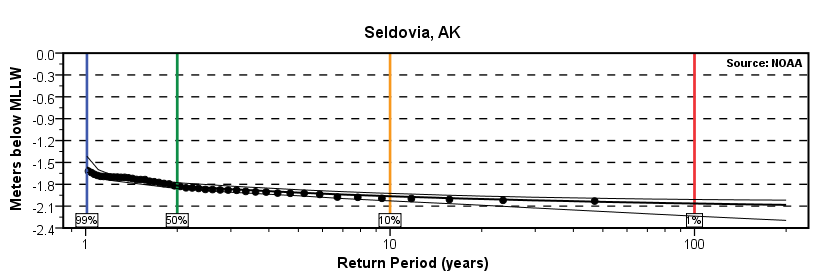### Annual Exceedance Probability Curves 9455500 Seldovia, AK

The annual exceedance probability curves with 95% confidence intervals shown below indicate the highest and lowest water levels as a function of return period in years. The dots indicate the annual highest or lowest water levels after the Mean Sea Level trend was removed, which were used to calculate the curves. The levels are in meters relative to the Mean Higher High Water (MHHW) or Mean Lower Low Water (MLLW) datums established by CO-OPS (1 foot = 0.3 meters). The horizontal position of the rightmost dot indicates the number of years of data used in the calculation.##### The 1% annual exceedance probability levels are 2.08 meters (6.82 feet) above Mean Higher High Water and 2.06 meters (6.76 feet) below Mean Lower Low Water.
The curves were calculated using the Extremes Toolkit software package which fits the three parameters of the Generalized Extreme Value (GEV) probability distribution function to annual maximum or annual minimum data using an iterative maximum likelihood estimation. The spread of the 95% confidence intervals depends on the variability of the source data and the length of the series used. The level of confidence in the exceedance probability level decreases with longer return periods and should always be used in conjunction with the confidence interval in the application of these data. The estimated uncertainty in the elevation of the tidal datums (MHHW and MLLW) is less than 0.01 meter.

##### Products available at 9455500 Seldovia, AK
Exceedance Probability Levels and Tidal Datums - Seldovia, AK - NOAA Tides & Currents